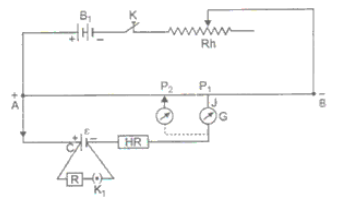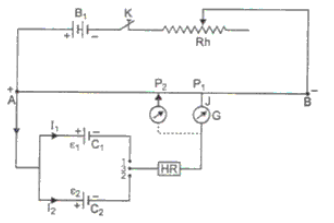1800-212-7858 (Toll Free)
9:00am - 8:00pm IST all days
8104911739

or

Thanks, You will receive a call shortly.
Customer Support

You are very important to us

For any content/service related issues please contact on this toll free number

022-62211530

Mon to Sat - 11 AM to 8 PM

# Draw a circuit diagram to compare the emf of two primary cells. Write the formula used. How can the sensitivity of a potentiometer be increased.ORDraw the circuit diagram of potentiometer which can be used to determine the internal resistance (r) of a given cell of emf (E). Explain with the help of this diagram a method to find the internal resistance of a primary cell. Give reasons why potentiometer is superior to voltmeter for determining internal resistance.

Asked by Topperlearning User 28th April 2015, 9:49 AM

Comparison of emf of two cells: First of all the ends of potentiometer are connected to a battery B1, key K and rheostat Rh such that the positive terminal of battery B1 is connected to end A of the wire. This completes the primary circuit.Now the positive terminals of the cells C1 and C2 whose emfs are to be compared are connected to A and the negative terminals to the jockey J through a two-way key and a galvanometer. This is the secondary circuit.

Method: (i) By closing key K, a potential difference is established and rheostat is so adjusted that when jockey J is made to touch at  ends A and B of wire, the deflection in galvanometer is on both sides. Suppose in this position the potential gradient is K.

(ii) Now plug is inserted between the terminals 1 and 3 so that cell C1 is included in the secondary circuit and jockey J is slided on the wire at P1 (say) to orbit the null point. The distance of P1 from A is measured. Suppose this length is l1i.e. AP1 = l1The emf of cell C1,.....(1)

(iii) Now plug is taken off between the terminals 1 and 3 and inserted in between the terminals  2 and 3 to bring cell C2 in the circuit. Jockey is slided on wire and null deflection P2 is noted. Suppose distance of P2 from A is l2 i,e, AP2 = l2The emf  of cell C2,.....(2)

Dividing (1) by (2), we getThus emfs of cells may be compared. Out of these cells if one standard cell, then the emf of other cell may be calucated.

Sensitivity: (i) To increase the sensitivity of measurement, the value of potential gradient k is kept least possible. Smaller the value of K, greater is the length (l) of the null deflection; and so greater will be the accuracy of measurement. That is way a very long wire is used in potentiometer.

OR

Determination of internal Resistance of potentiometer:

Circuit: A battery B1, a rheostat (Rh) and a key K is connected across the ends A and B of the potentiometer wire such that positive terminal of battery is connected to points A. This completes the primary ciruuit.

Now the give cell C is connected such that its positive terminal is connected to A and negative terminal to jockey J through a galvanometer. A resistance box (R) and a key K1 are connected across the cell. This completes the secondary circuit.Method: (i) Initially key K is close and a potential difference is applied across the wire AB. Now rheostat (Rh) is so adjusted that on touching the jockey J at ends A and B of potentiometer wire, the deflection in the galvanometer is on both sides. Suppose that in this position the potential gradient on the wire is K.

(ii) Now key K1 is kept open and the position of null deflection is obtained by sliding and pressing the jockey on the wire. Let this position be P1 and AP1 = l1

In this situation the cell is in open circuit, therefore the terminal potential difference will be equal to the emf of cell, ie.,

emf......(1)

(iii) Now a suitable resistance R is taken in the resistance box and key K1 is close. Again, the position of null point is obtained on the wire by using jockey J. Let this position on wire be P2 and AP2 = l2.

In this situation the cell is in closed circuit, therefor the terminal potential difference

(V) of cell will be equal to the potential difference across external resistance R i.e.,

V = Kl2                                           ......(2)

Dividing (1) by (2), we getInternal resistance of cell r =From this formula r may be calculated.

Superiority of potentiometer to voltmeter.

(i) If the process of measuring emf of a cell by potentiometer in the null point position, on current flows in the cell circuit, so cell remains in open circuit. Hence, we obtain the actual value of emf. Thus we may say that a potentiometer is equivalent to a voltmeter of infinite resistance (i.e., ideal voltmeter).

(ii) For measuring the emf by a voltmeter, the deflection in the voltmeter is noted. There may be some error in reading the deflection. On the other hand for measuring the emf by a potentiometer, only null is to read which involves less error.

Thus a potentiometer is superior to a voltmeter.

Answered by Expert 28th April 2015, 11:49 AM
• 1
• 2
• 3
• 4
• 5
• 6
• 7
• 8
• 9
• 10

You have rated this answer /10